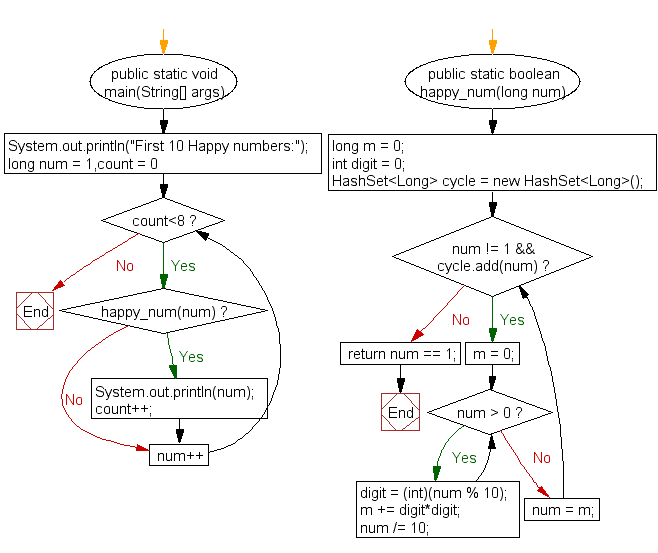﻿ Java exercises: Find and print the first 10 happy numbers - w3resource# Java Exercises: Find and print the first 10 happy numbers

## Java Numbers: Exercise-9 with Solution

Write a Java program to find and print the first 10 happy numbers.

Happy number: Starting with any positive integer, replace the number by the sum of the squares of its digits, and repeat the process until the number equals 1, or it loops endlessly in a cycle which does not include 1.

Example: 19 is a happy number
12 + 92=82
82 + 22=68
62 + 82=100
12 + 02 + 02=1

Pictorial Presentation:Sample Solution:

Java Code:

``````import java.util.HashSet;
public class Example9 {
public static void main(String[] args){
System.out.println("First 10 Happy numbers:");
for(long num = 1,count = 0;count<8;num++){
if(happy_num(num)){
System.out.println(num);
count++;
}
}
}
public static boolean happy_num(long num){
long m = 0;
int digit = 0;
HashSet<Long> cycle = new HashSet<Long>();
m = 0;
while(num > 0){
digit = (int)(num % 10);
m += digit*digit;
num /= 10;
}
num = m;
}
return num == 1;
}
}
```
```

Sample Output:

```First 10 Happy numbers:
1
7
10
13
19
23
28
31
```

Flowchart:Java Code Editor:

What is the difficulty level of this exercise?

﻿

## Java: Tips of the Day

Array vs ArrayLists:

The main difference between these two is that an Array is of fixed size so once you have created an Array you cannot change it but the ArrayList is not of fixed size. You can create instances of ArrayLists without specifying its size. So if you create such instances of an ArrayList without specifying its size Java will create an instance of an ArrayList of default size.

Once an ArrayList is full it re-sizes itself. In fact, an ArrayList is internally supported by an array. So when an ArrayList is resized it will slow down its performance a bit as the contents of the old Array must be copied to a new Array.

At the same time, it's compulsory to specify the size of an Array directly or indirectly while creating it. And also Arrays can store both primitives and objects while ArrayLists only can store objects.

Ref: https://bit.ly/3o8L2KH